Monday 08th August 2022CBSE Guess > Papers > Important Questions > Class XI > 2010 > Maths > Maths By Mr. Shivankar Gupta CBSE CLASS XI
Q. 1. Write the first five term of each of the  sequence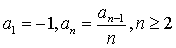Q. 2. In an A.P. the first term is 2 and the sum of first five terms is one fourth of the sum of next five term. Show that 20th term is --112? Q. 3. The sum of n terms of two arithmetic progressions are in the ratio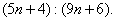Find the ratio of their 18th terms. Q. 4. Between 1 and 31, m numbers have been inserted in such a way that the resulting sequence is an A.P. and the ratio of 7th and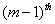numbers is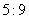. Find the value of m. Q. 5. The difference between any two consecutive interior angles of a polygon is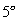. If the smallest angle is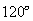. Find the number of sides of the polygon. Q. 6. If the sum of first p terms of an A.P. is equal to the sum of first q terms, then find the sum of first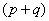term.. Q. 7. If the sum of n terms of an A.P. is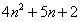. Find the series and its nth term. Q. 8. Find the sum of all two digit numbers which when divided by 4 , yields 1 as remainder.   Paper By Mr. Shivankar Gupta Email Id : [email protected] Ph No. : 9410407427